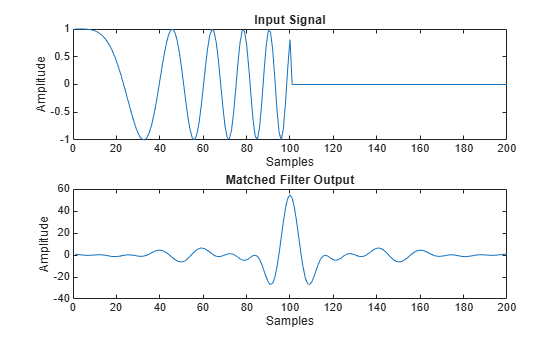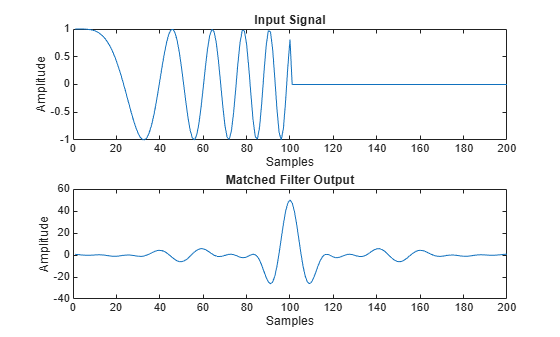Documentation

phased.MatchedFilter

Matched filter

Description

The MatchedFilter object implements matched filtering of an input signal.

To compute the matched filtered signal:

1. Define and set up your matched filter. See Construction.

2. Call step to perform the matched filtering according to the properties of phased.MatchedFilter. The behavior of step is specific to each object in the toolbox.

Note

Starting in R2016b, instead of using the step method to perform the operation defined by the System object™, you can call the object with arguments, as if it were a function. For example, y = step(obj,x) and y = obj(x) perform equivalent operations.

Construction

H = phased.MatchedFilter creates a matched filter System object, H. The object performs matched filtering on the input data.

H = phased.MatchedFilter(Name,Value) creates a matched filter object, H, with each specified property Name set to the specified Value. You can specify additional name-value pair arguments in any order as (Name1,Value1,...,NameN,ValueN).

Properties

CoefficientsSource

Source of matched filter coefficients

Specify whether the matched filter coefficients come from the Coefficients property of this object or from an input argument in step. Values of this property are:

 'Property' The Coefficients property of this object specifies the coefficients. 'Input port' An input argument in each invocation of step specifies the coefficients.

Default: 'Property'

Coefficients

Matched filter coefficients

Specify the matched filter coefficients as a column vector. This property applies when you set the CoefficientsSource property to 'Property'. This property is tunable.

Default: [1;1]

SpectrumWindow

Window for spectrum weighting

Specify the window used for spectrum weighting using one of 'None', 'Hamming', 'Chebyshev', 'Hann', 'Kaiser', 'Taylor', or 'Custom'. Spectrum weighting is often used with linear FM waveform to reduce the sidelobes in the time domain. The object computes the window length internally, to match the FFT length.

Default: 'None'

CustomSpectrumWindow

User-defined window for spectrum weighting

Specify the user-defined window for spectrum weighting using a function handle or a cell array. This property applies when you set the SpectrumWindow property to 'Custom'.

If CustomSpectrumWindow is a function handle, the specified function takes the window length as the input and generates appropriate window coefficients.

If CustomSpectrumWindow is a cell array, then the first cell must be a function handle. The specified function takes the window length as the first input argument, with other additional input arguments if necessary, and generates appropriate window coefficients. The remaining entries in the cell array are the additional input arguments to the function, if any.

Default: @hamming

SpectrumRange

Spectrum window coverage region

Specify the spectrum region on which the spectrum window is applied as a 1-by-2 vector in the form of [StartFrequency EndFrequency] (in hertz). This property applies when you set the SpectrumWindow property to a value other than 'None'.

Note that both StartFrequency and EndFrequency are measured in baseband. That is, they are within [-Fs/2 Fs/2], where Fs is the sample rate that you specify in the SampleRate property. StartFrequency cannot be larger than EndFrequency.

Default: [0 1e5]

SampleRate

Coefficient sample rate

Specify the matched filter coefficients sample rate (in hertz) as a positive scalar. This property applies when you set the SpectrumWindow property to a value other than 'None'.

Default: 1e6

SidelobeAttenuation

Window sidelobe attenuation level

Specify the sidelobe attenuation level (in decibels) of a Chebyshev or Taylor window as a positive scalar. This property applies when you set the SpectrumWindow property to 'Chebyshev' or 'Taylor'.

Default: 30

Beta

Kaiser window parameter

Specify the parameter that affects the Kaiser window sidelobe attenuation as a nonnegative scalar. Please refer to kaiser for more details. This property applies when you set the SpectrumWindow property to 'Kaiser'.

Default: 0.5

Nbar

Number of nearly constant sidelobes in Taylor window

Specify the number of nearly constant level sidelobes adjacent to the mainlobe in a Taylor window as a positive integer. This property applies when you set the SpectrumWindow property to 'Taylor'.

Default: 4

GainOutputPort

Output gain

To obtain the matched filter gain, set this property to true and use the corresponding output argument when invoking step. If you do not want to obtain the matched filter gain, set this property to false.

Default: false

Methods

 step Perform matched filtering
Common to All System Objects
release

Allow System object property value changes

Examples

expand all

Construct a matched filter for a linear FM waveform.

waveform = phased.LinearFMWaveform('PulseWidth',1e-4,'PRF',5e3);
x = waveform();
filter = phased.MatchedFilter( ...
'Coefficients',getMatchedFilter(waveform));
y = filter(x);
subplot(2,1,1),plot(real(x))
xlabel('Samples')
ylabel('Amplitude')
title('Input Signal')
subplot(2,1,2),plot(real(y))
xlabel('Samples')
ylabel('Amplitude')
title('Matched Filter Output')Apply a matched filter, using a Hamming window to do spectrum weighting.

waveform = phased.LinearFMWaveform('PulseWidth',1e-4,'PRF',5e3);
x = waveform();
filter = phased.MatchedFilter( ...
'Coefficients',getMatchedFilter(waveform), ...
'SpectrumWindow','Hamming');
y = filter(x);
subplot(2,1,1)
plot(real(x))
xlabel('Samples')
ylabel('Amplitude')
title('Input Signal')
subplot(2,1,2)
plot(real(y))
xlabel('Samples')
ylabel('Amplitude')
title('Matched Filter Output')Apply a matched filter, using a custom Gaussian window for spectrum weighting.

waveform = phased.LinearFMWaveform('PulseWidth',1e-4,'PRF',5e3);
x = waveform();
filter = phased.MatchedFilter( ...
'Coefficients',getMatchedFilter(waveform), ...
'SpectrumWindow','Custom', ...
'CustomSpectrumWindow',{@gausswin,2.5});
y = filter(x);
subplot(2,1,1)
plot(real(x))
xlabel('Samples')
ylabel('Amplitude')
title('Input Signal')
subplot(2,1,2)
plot(real(y))
xlabel('Samples')
ylabel('Amplitude')
title('Matched Filter Output')Algorithms

The filtering operation uses the overlap-add method.

Spectrum weighting produces a transfer function

$H\text{'}\left(F\right)=w\left(F\right)H\left(F\right)$

where w(F) is the window and H(F) is the original transfer function.

For further details on matched filter theory, see or .

References

 Richards, M. A. Fundamentals of Radar Signal Processing. New York: McGraw-Hill, 2005.

 Skolnik, M. Introduction to Radar Systems, 3rd Ed. New York: McGraw-Hill, 2001.